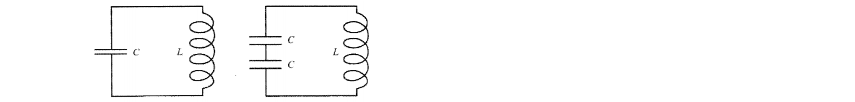# Problem: The circuit on the left has an oscillation frequency of 400 Hz. The frequency of the circuit on the right is? a. 200 Hz b. 282.8 Hz c. 400 Hz d. 565.7 Hz e. 800 Hz

###### FREE Expert Solution
85% (244 ratings)
###### Problem Details

The circuit on the left has an oscillation frequency of 400 Hz. The frequency of the circuit on the right is?

a. 200 Hz

b. 282.8 Hz

c. 400 Hz

d. 565.7 Hz

e. 800 HzFrequently Asked Questions

What scientific concept do you need to know in order to solve this problem?

Our tutors have indicated that to solve this problem you will need to apply the LC Circuits concept. You can view video lessons to learn LC Circuits. Or if you need more LC Circuits practice, you can also practice LC Circuits practice problems.

How long does this problem take to solve?

Our expert Physics tutor, Juan took 2 minutes and 7 seconds to solve this problem. You can follow their steps in the video explanation above.

What professor is this problem relevant for?

Based on our data, we think this problem is relevant for Professor Papavasiliou's class at VT.